Easy-to-Read Tableau Reference Guide on Calculated Fields

# Easy-to-Read Tableau Reference Guide on Calculated Fields – Number FunctionsIt’s not possible for everyone to ace in Tableau, at least not yet. Tableau is a versatile data visualization application that facilitates users to examine structured data virtually, while displaying information in several interactive graphic perspectives. Though it’s very easy to use and a lot of individuals use Tableau Desktop for creating data visualizations, it churns out best results when employed by expert hands.

Thus, to help Tableau stalwarts, we’re here with a set of blogs on Tableau Essentials that will help you dig into the basics of using this powerful data visualization software, especially Desktop versions 8.1 and 8.2. This blog is a continuation of the Tableau blog on Logical Functions. It was part I and this one, which exclusively sheds light on Number Functions is part II. Here, we will deeply focus on another group of functions implemented for Tableau’s calculated fields. Scroll below to get started…

First, open the Calculated Fields window, right-click on the sidebar (Data window) and choose Create Calculated Field: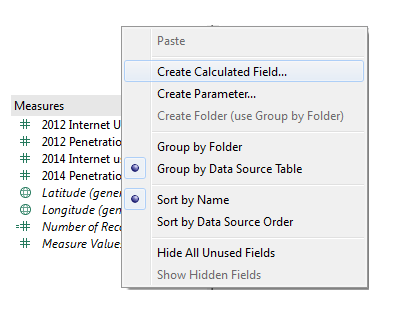Now, in the Calculated Field Window, choose Number from the Functions drop-down menu: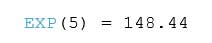#### LN FUNCTION

LN(number)

It returns the natural log of the number. Now, if the numbers appear to be less than or equal to zero, the function tends to return NULL.

For an example,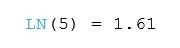#### LOG FUNCTION

LOG(number,[base])

LOG brings back the log of the number for a given base. In case, there’s no base, the function will use base 10 by default.

For an example,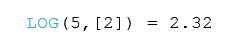#### PI FUNCTION

PI()

It helps return the numeric constant of PI.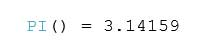#### POWER FUNCTION

POWER(number, function)

This function increases the number to the defined power.

For an example,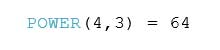This is a superb function to convert numbers from degrees to radians.#### ROUND FUNCTION

ROUND(number,[decimals])

Use this function to round off any number to the nearest integer or to a particular number of decimal places.

For an instance,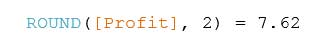#### SIGN FUNCTION

SIGN(number)

This function brings back the sign of a number.

In case of positive numbers, it returns a 1.

For zero, it returns a 0.

For negative numbers, the function returns a -1.

For an example,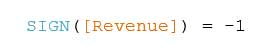#### SQRT FUNCTION

SQRT(number)

It returns the square root of a number.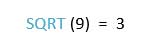#### SQUARE FUNCTION

SQUARE(number)

This function returns the square of the number.

For an instance,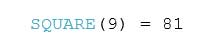#### ZN FUNCTION

ZN(expression)

The specialty of ZN function is that it evaluates any expression.

If the function is NULL, it will return a value of 0, and if not, the expression is returned as before.

For example,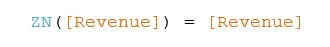STATISTICAL

#### MAX FUNCTION

MAX(number, number)

This function returns the maximal of two expressions for each record or an expression throughout all records. However, the two statements have to be the same type. If one or the other argument turns NULL, the function returns a value of NULL.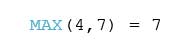#### MIN FUNCTION

MIN(number, number)

Just like MAX function, MIN function too returns the minimal of an expression across the records or minimal of two expressions for a particular record. The two arguments must be similar in type. Also, if one or the other arguments hold NULL, MIN returns a value NULL.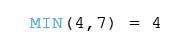TRIGONOMETRIC

#### ACOS FUNCTION

ACOS(number)

ACOS function returns the arc cosine of the number and the outcome is in radians.

Take a look,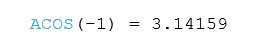#### ASIN FUNCTION

ASIN(number)

This function returns the arc sine of the number. And as usual the outcome is in radians.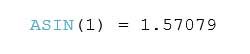#### ATAN FUNCTION

ATAN(number)

It returns the arc tangent of any number, and as usual the outcomes is in radians.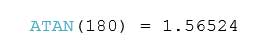#### ATAN2 FUNCTION

ATAN2(y number, x number)

It’s quite similar to the previous ATAN FUNCTION, except it’s used for two given numbers. Otherwise, all remains same.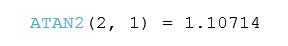#### COS FUNCTION

COS(number)

Cos returns the cosine of an angle. Just mention the angle in radians.

For example,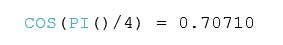#### COT FUNCTION

COT(number)

COT FUNCTION returns the cotangent of an angle. Marking of angles in radians is important.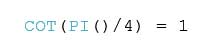#### SIN FUNCTION

SIN(number)

This function returns the sine of an angle. For example,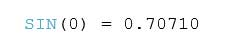#### TAN FUNCTION

TAN(number)

TAN FUNCTION returns the tangent of an angle. You just need to mention the angle in radians and that’s it.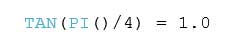Typically, it all depends on the nature of your business; if it needs, you have to go through Number Functions routinely, otherwise not. Now, if you really have to use them then peruse over Tableau course details at DexLab Analytics. Being a premier Tableau training institute in Gurgaon, DexLab will offer a whole new layer of insight into Tableau Essentials.

The article has been sourced from – https://interworks.com/blog/ccapitula/2015/04/07/tableau-essentials-calculated-fields-number-functions